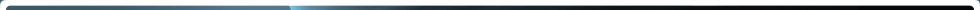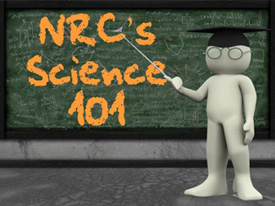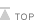# What is Matter ?

Printable Version

## The Nuclear Regulatory Commission's Science 101: What is Matter?Everything that exists is made up of matter. Matter has two fundamental properties: volume and mass. Volume simply refers to the space an object takes up. Depending on the physical state of an object, there are a couple ways to measure volume. If we are trying to measure the volume of a box, for instance, we would multiply the length of the box by its height and by its width.

Let's say that we have a box with the following dimensions: length = 3 meters ("m"), height = 4m, and width = 5m. Based on those dimensions, our box would have a volume of 60m3 (3m x 4m x 5m = 60m3). That is, again, a measure (in cubic meters) of how much three-dimensional space our box takes up.

If, on the other hand, our object was a liquid, we could use a graduated cylinder (a scientific measuring cup) to measure the volume of our object. This measure would be reported in liters. Again, a liter is just a measure of how much space a liquid takes up. For example, you can purchase soda in 2-liter bottles.

Since we've been talking a little about measurements, it might make sense at this point to distinguish between quantity and units. Thinking again about our example, the quantity we are trying to measure is volume. The unit we use to report this measurement is in liters or cubic meters.

.Now, let's now talk about the other fundamental property of matter — mass. When we talk about mass, we are referring to how much "stuff" is in an object. To illustrate this, think about two pieces of candy, both of the same kind and both the same size, however one of them is hollow. The candy that isn't hollow has more mass compared to the hollow candy. Given that we often use scales to measure mass, you might think that mass and weight are the same thing. But they aren't. Mass is the measure of matter in a particular object. No matter where that object is in the vast universe, it will have the same mass.

Weight, on the other hand, is a measure of how much gravitational force is exerted on an object. While the weight of an object is proportional to its mass (the more mass of an object the more it will weigh), gravity varies according by where you are in the universe or even where you are on Earth—you actually weigh more, because there is a higher gravitational force, on the poles than you would at the equator. So, while an object will have a particular weight here on Earth, it will not have the same weight on the moon. It would, however, have the same mass both places.

Now that we can determine if something is matter (if it has volume and mass), we can use another measurement, density, to determine what kind of matter a substance is. Density is the ratio of how much mass is in an object compared to the volume of that object. Density is calculated by dividing an object's mass by its volume.

Think back to our box with a volume of 60m3. Let's say that our box has a mass of 240 grams (g). If that were the case, the density of our box would be 4 g/m3 (240g / 60m3). Density is nothing more than a way of stating how much matter fits within a particular volume. Using the two pieces of candy, while both have the same size (or volume), the solid one has more mass when compared to the hollow one and, as such, the solid candy is more dense (more matter in a particular volume) than the hollow candy.

Because the density of a particular substance (something with a defined composition, such as pure copper), is the same for all pieces of that substance, regardless of size, density is often useful in determining the identity of a particular object. Once we've calculated the density of an object, we can compare that value to the known densities for substances to determine what substance we believe the object is.

The U.S. Nuclear Regulatory Commission is an independent federal government agency responsible for regulating the commercial use of nuclear materials. This document is free of copyright and can be reproduced for educational purposes.Page Last Reviewed/Updated Friday, October 19, 2018

#### Home